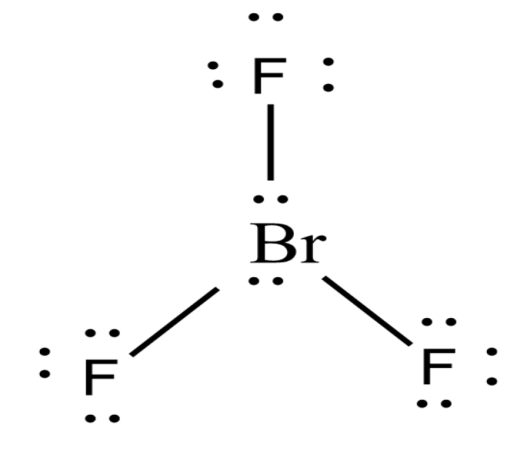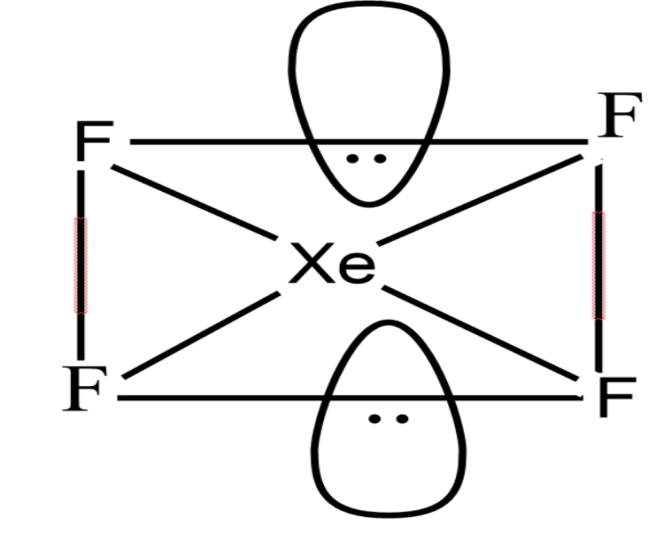Write the structures of the following:(i) $Br{{F}_{3}}$(ii) $Xe{{F}_{4}}$Verified
146.4k+ views
Hint: Prediction of structures of compounds can be done through the VSEPR (Valence-shell electron-pair repulsion) model. This model is based on the idea that the lowest-energy arrangement for a compound is the one in which in its electron pairs (bonding and non-bonding) are as far as possible.

(i) $Br{{F}_{3}}$
-The VSEPR model can help us predict the structure of nearly any molecule or polyatomic ion in which the central atom is a non-metal, as well as the structures of many molecules and polyatomic ions with a central metal ion.
-Bromine trifluoride ($Br{{F}_{3}}$) is an interhalogen compound of straw-colored liquid with a pungent odor.
- $Br{{F}_{3}}$ contains three bonded and two bonded electron domains giving a T-shaped and planar molecular geometry. The distance of axial fluorine from the bromine is 1.81 $A{}^\circ$and the equatorial fluorine is 1.72 $A{}^\circ$ . The angle between axial fluorine and the equatorial fluorine is slightly smaller than 90${}^\circ$ to 86.2 ${}^\circ$. The Lewis dot structure of Bromine trifluoride is--The formula used to know the molecular shape is
Number of electrons $=\frac{1}{2}\left[ V+N-C+A \right]$
Where V is the number of valence electrons present in the central atom
N is the number of monovalent atoms bonded to the central atom
C is the charge of the cation
A is the charge of the anion
Now, applying the values,
Number of electrons$=\frac{1}{2}\left[ 7+3-0+0 \right]=5$
Since the number of electrons is 5 which means that it has $s{{p}_{3}}d$hybridization and the molecule will be trigonal pyramidal.
But, there are three bond pairs and two lone pairs. The two lone pairs will occupy the equatorial position, due to which it will cause the distraction of the molecule hence it has a distorted ‘T’ shape structure.

(ii) $Xe{{F}_{4}}$
-It was the first discovered binary compound of a noble gas.
-Xenon tetrafluoride is a colorless crystalline substance with a square planar geometry.
- The formula used to know the molecular shape is
Number of electrons $=\frac{1}{2}\left[ V+N-C+A \right]$
Where V is the number of valence electrons present in the central atom
N is the number of monovalent atoms bonded to the central atom
C is the charge of the cation
A is the charge of the anion
Now, applying the values,
Number of electrons $=\frac{1}{2}\left[ 8+4-0+0 \right]=6$
Since the number of electrons is 6 which means that it has $s{{p}^{3}}d$ with square planar geometry.$Xe{{F}_{4}}$ have two lone pairs of electrons. The bond pair electrons and lone pair electrons experience repulsion between them hence they acquire a stable state. The lone pair of Xenon lies in the perpendicular plane in an octahedral arrangement, hence it shows square planar geometry.

Note: Be careful while counting the number of electrons for calculations. One important thing to note is that coordination number 4 can show both tetrahedral as well as square planar geometry. If the central metal atom is showing $ds{{p}^{2}}$ hybridization, it will exhibit square planar geometry. If the central metal atom is $s{{p}^{3}}$ hybridized, it will exhibit tetrahedral geometry.